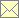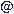Laser Doppler Data Processing Techniques - Local Normalization

 Home Scope Literature Data Programs Techniques Links Impressum Data Protection Policy Data Basewebmasternambisde 12.3.2018

Local Normalization is a technique, where the correlation coefficient $ϱ$ is derived from the primary correlation estimate by normalization with the two correlation estimates of the appropriate self-products

$ϱ ( τ ) = ∑ i ∑ j ≠ i ( | t j - t i - τ | < Δ τ 2 ) w i w j u i u j [ ∑ i ∑ j ≠ i ( | t j - t i - τ | < Δ τ 2 ) w i w j u i 2 ] [ ∑ i ∑ j ≠ i ( | t j - t i - τ | < Δ τ 2 ) w i w j u j 2 ]$

where $u i$ are understood as values with subtracted mean. However, due to noise, the denominator has a bias, while the numerator has not. Therefore, the correlation coefficient should be de-normalized by an estimate of the variance, which is also affected by noise.

$R ( τ ) = ϱ ( τ ) ⋅ ∑ i w i u i 2 ∑ i w i$

This estimate is not affected by noise, while the estimation variance decreases compared with the primary correlation estimate. If needed, a re-normalization can finally be done, which then is not biased due to noise.

$ϱ ( τ ) = R ( τ ) R (0)$

original papers:

• Tummers M J, Passchier D M and Aswatha Narayana P A (1995): LDA measurements of time- and spatial correlation functions in an adverse pressure gradient wake. Proc. 6th Int. Conf. on Laser Anemom. - Adv. and Appl., Hilton Head Island, South Corolina, USA, FED-Vol. 229, Laser Anemometry, ASME 1995, 347-354
• van Maanen H R E and Tummers M J (1996): Estimation of the Auto Correlation Function of Turbulent Velocity Fluctuations using the Slotting Technique with Local Normalisation. Proc. 8th Int. Symp. on Appl. of Laser Techn. to Fluid Mech., Lisbon, Portugal, paper 36.4

combined with fuzzy slotting:

combined with fuzzy slotting and forward-backward arrival-time weighting:

adapted to direct spectral estimation: